# What is Coherent Lightwave Communication System?

### What is Intensity Modulation with Direct Detection (IM/DD)?

Current fiber optic lightwave communication systems are based on a simple digital transmission scheme in which an electrical bit stream is used to modulate the intensity of the optical carrier, and the optical signal is detected directly at a photodiode to convert it to the original digital signal in the electrical domain.

Such a scheme is referred to as intensity modulation with direct detection (IM/DD).

### What is Coherent Optical Communication?

In contrast to intensity modulation with direct detection (IM/DD), many alternative schemes, well known in the context of radio and microwave communication systems, transmit information by modulating the frequency or the phase of the optical carrier and detect the transmitted signal by using homodyne or or heterodyne detection techniques.

Since phase coherence of the optical carrier plays an important role in the implementation of such schemes, they are referred to as coherent communication techniques, and the fiber optic communication systems based on them are called coherent lightwave systems.

Coherent communication techniques were explored during the 1980s, and many field trials established their feasibility by 1990.

### Why Do We Need Coherent Lightwave Systems?

The motivation behind using the coherent communication techniques is twofold.

1) Receiver sensitivity can be improved by up to 20dB compared with IM/DD systems. Such an improvement permits much longer transmission distance (up to an additional 100km near 1.55um) for the same amount of transmitter power.

2) The use of coherent detection allows an efficient use of fiber bandwidth. Many channels can be transmitted simultaneously over the same fiber by using frequency-division multiplexing (FDM) with a channel spacing as small as 1-10 GHz.

### Basic Concepts Behind Coherent Lightwave Systems

#### 1. Local Oscillator

The basic idea behind coherent lightwave system is to mix the received signal coherently with a continuous-wave (CW) optical field before it is incident on the photodetector (as shown in figure 1 below).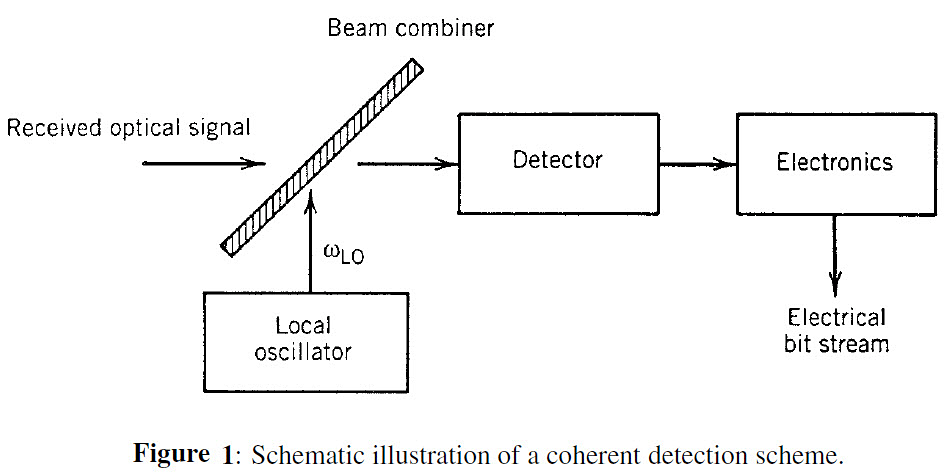The continuous wave field is generated locally at the receiver using a narrow-linewidth laser, called the local oscillator (LO), a term borrowed from the radio and microwave literature.

To see how mixing of the received signal with a local oscillator can improve the receiver performance, let us write the optical signal using complex notation as

where ωo is the carrier frequency, As is the amplitude, and Φs is the phase.

The optical field associated with the local oscillator is given by a similar expression,

(Equation 1.2)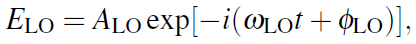where ALO, ωLO, and ΦLO represent the amplitude, frequency, and phase of the the local oscillator, respectively

The scalar notation is used for both Es and ELO after assuming that the two fields are identically polarized (polarization-mismatch issues can be discussed later).

Since a photodetector responds to the optical intensity, the optical power incident at the photodetector is given by

P = K|Es+ELO|2

where K is a constant of proportionality.

Using Eqs. (1.1 and 1.2), we get

(Equation 1.3)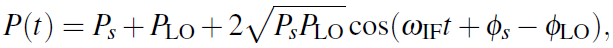where

(Equation 1.4)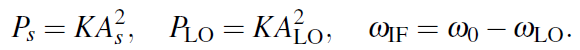The frequency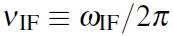is known as the intermediate frequency (IF).

When ω0  ωLO, the optical signal is demodulated in two stages, its carrier frequency is first converted to an intermediate frequency νIF (typically 0.1-5GHz) before the signal is demodulated to the baseband.

It is not always necessary to use an intermediate frequency. In fact, there are two different coherent detection techniques to choose from, depending on whether or not νIF equals zero. They are known as homodyne and heterodyne detection techniques.

#### 2. Homodyne Detection

In this coherent-detection technique, the local-oscillator frequency ωLO is selected to coincide with the signal-carrier frequency ω0 so that ωIF = 0.

From Equation 1.3, the photocurrent (I=RP, where R is the detector responsivity) is given by

(Equation 1.5)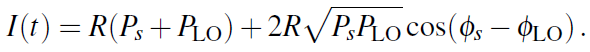Typically, PLO >> Ps, and Ps + PLO ≈ PLO.

The last term in Equation 1.5 contains the information transmitted and is used by the decision circuit. Consider the case in which the local-oscillator phase is locked to the signal phase so that Φs = ΦLO. The homodyne signal is then given by

(Equation 1.6)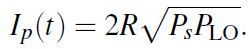The main advantage of homodyne detection is evident from equation 1.6 if we note that the signal current in the direct-detection case is given by Idd(t) = RPs(t). Denoting the average optical power by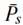, the average electrical power is increased by a factor of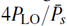with the use of homodyne detection.

Since PLO can be made much larger than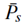, the power enhancement can exceed 20dB. Although shot noise is also enhanced, it is shown that homodyne detection improves the signal-to-noise ratio (SNR) by a large factor.

Another advantage of coherent detection is evident from Equation 1.5. Because the last term in this equation contains the signal phase explicitly, it is possible to transmit information by modulating the phase or frequency of the optical carrier. Direct detection does not allow phase or frequency modulation, as all information about the signal phase is lost.

A disadvantage of homodyne detection also results from its phase sensitivity. Since the last term in equation 1.5 contains the local-oscillator phase ΦLO explicitly, clearly ΦLO should be controlled.

Ideally, Φs and ΦLO should stay constant except for the intentional modulation of Φs. In practice, both Φs and ΦLO fluctuate with time in a random manner. However, their difference Φs – ΦLO can be forced to remain nearly constant through an optical phase-locked loop.

The implementation of such as loop is not simple and makes the design of optical homodyne receivers quite complicated. In addition, matching of the transmitter and local-oscillator frequencies puts stringent requirements on the two optical sources. These problems can be overcome by the use of heterodyne detection, as discussed next.

#### 3. Heterodyne Detection

In the case of heterodyne detection the local-oscillator frequency ωLO is chosen to differ from the signal-carrier frequency ω0 such that the intermediate frequency ωIF is in the microwave region (νIF ~ 1 GHz). Using Equation 1.3 together with I = RP, the photocurrent is now given by

(Equation 1.7)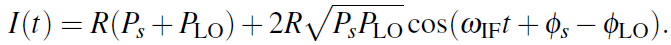Since PLO >> Ps in practice, the direct-current (dc) term is nearly constant and can be removed easily using bandpass filters. The heterodyne signal is then given by the alternating-current (ac) term in equation 1.7 or by

(Equation 1.8)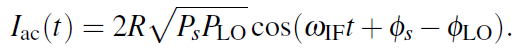Similar to the case of homodyne detection, information can be transmitted through amplitude, phase, or frequency modulation of the optical carrier. More importantly, the local oscillator still amplifies the received signal by a large factor, thereby improving the SNR.

However, the SNR improvement is lower by a factor of 2 (or by 3dB) compared with the homodyne case. This reduction is referred to as the heterodyne-detection penalty.

The origin of the the 3dB penalty can be seen by considering the signal power (proportional to the square of the current). Because of the ac nature of Iac, the average signal power is reduced by a factor of 2 when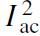is averaged over a full cycle at the intermediate frequency (recall that the average of cos2θ over θ is 1/2).

The advantage gained at the expense of the 3dB penalty is that the receiver design is considerably simplified because an optical phase-locked loop is no longer needed.

Fluctuations in both Φs and ΦLO still need to be controlled using narrow-linewidth semiconductor lasers for both optical sources. However, the linewidth requirements are quite moderate when an asynchronous demodulation scheme is used. This feature makes the heterodyne-detection scheme quite suitable for practical implementation in coherent lightwave systems.

#### 4. Signal-to-Noise Ratio

The advantage of coherent detection for lightwave systems can be made more quantitative by considering the SNR of the receiver current.

The receiver current fluctuates because of shot noise and thermal noise. The variance σ2 of current fluctuation is obtained by adding the two contributions so that

(Equation 1.9)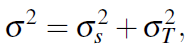where

(Equation 1.10)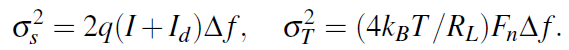The current I in equation 1.10 is the total photocurrent generated at the detector and is given by equation 1.5 or 1.7, depending on whether homodyne or heterodyne detection is employed. In practice, PLO >> Ps, and I in equation 1.10 can be replaced by the dominant term RPLO for both cases.

The SNR is obtained by dividing the average signal power by the average noise power. In the heterodyne case, it is given by

(Equation 1.11)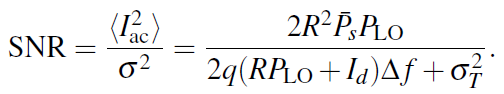In the homodyne case, the SNR is larger by a factor of 2 if we assume that Φs = ΦLO in Equation 1.5.

The main advantage of coherent detection can be seen from Equation 1.11. Since the local-oscillator power PLO can be controlled at the receiver, it can be made large enough that the receiver noise is dominated by shot noise. More specifically,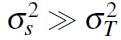when

(Equation 1.12)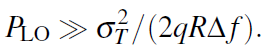Under the same conditions, the dark-current contributions to the shot noise is negligible (Id << RPLO). The SNR is then given by

(Equation 1.13)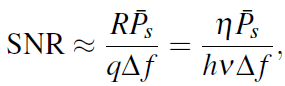where R = ηq/hν.

The use of coherent detection allows one to achieve the shot-noise limit even for p-i-n receivers whose performance is generally limited by thermal noise. Moreover, in contrast with the case of avalanche photodiode (APD) receivers, this limit is realized without adding any excess shot noise.

It is useful to express the SNR in terms of the number of photons, Np, received within a single bit. At the bit rate B, the signal power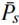is related to Np as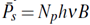. Typically Δf ≈ B/2. By using these values of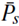and Δf in equation 1.13, the SNR is given by a simple expression

(Equation 1.14)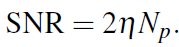In the case of homodyne detection, SNR is larger by a factor of 2 and is given by SNR = 4ηNp. There are more discussions regarding the dependence of the BER on SNR and shows how receiver sensitivity is improved by the use of coherent detection.

### Modulation Formats

As we said earlier, an important advantage of using the coherent detection techniques is that both the amplitude and the phase of the received optical signal can be detected and measured. This feature opens up the possibility of sending information by modulating either the amplitude, or the phase, or the frequency of an optical carrier.

In the case of digital communication systems, the three possibilities give rise to three modulation formats known as amplitude-shift keying (ASK), phase-shift keying (PSK), and frequency-shift keying (FSK).

Figure 2 below shows schematically the three modulation formats for a specific bit pattern.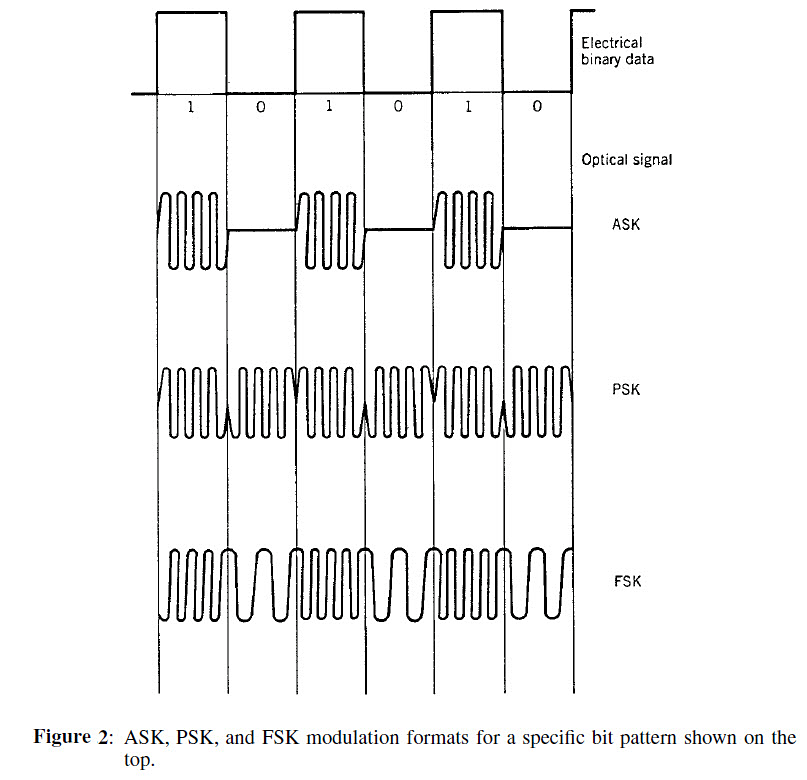The electric field associated with an optical signal can be written as

(Equation 2.1)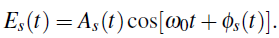In the case of ASK format, the amplitude As is modulated while keeping ω0 and Φs constant. For binary digital modulation, As takes one of two fixed values during each bit period, depending on whether 1 or 0 bit is being transmitted.

In most practical situations, As is set to zero during transmission of 0 bits. The ASK format is then called on-off keying (OOK) and is identical with the modulation scheme commonly used for noncoherent (IM/DD) digital lightwave systems.

The implementation of ASK for coherent systems differs from the case of direct-detection systems in one important aspect. Whereas the optical bit stream for direct-detection systems can be generated by modulating a light-emitting diode (LED) or a semiconductor laser directly, external modulation is necessary for coherent communication systems.

The reason behind this necessity is related to phase changes that invariably occur when the amplitude As (or the power) is changed by modulating the current applied to a semiconductor laser. For IM/DD systems, such unintentional phase changes are not seen by the detector (as the detector responds only to the optical power) and are not of major concern except for the chirp-induced power penalty.

The situation is entirely different in the case of coherent systems, where the detector response depends on the phase of the received signal. The implementation of ASK format for coherent systems requires the phase Φs to remain nearly constant. This is achieved by operating the semiconductor laser continuously at a constant current and modulating its output by using an external modulator.

Since all external modulators have some insertion losses, a power penalty incurs whenever an external modulator is used; it can be reduced to below 1dB for monolithically integrated modulators.

A commonly used external modulator makes use of LiNbO3 waveguides in a Mach-Zehnder (MZ) configuration. The performance of external modulators is quantified through the on-off ratio (also called extinction ratio) and the modulation bandwidth. LiNbO3 modulators provide an on-off ratio in excess of 20 and can be modulated at speeds up to 75GHz. The driving voltage is typically 5V but can be reduced to 3V with a suitable design.

Other materials can also be used to make external modulators. For example, a polymeric electro-optic MZ modulator required only 1.8V for shifting the phase of a 1.55um signal by π in one of the arms of the MZ interferometer.

Electroabsorption modulators, made using semiconductors, are often preferred because they do not require the use of an interferometer and can be integrated monolithically with the laser. Optical transmitters with an integrated electroabsorption modulator capable of modulating at 10 Gb/s were available since 1999 and are used routinely for IM/DD lightwave systems. Such integrated modulators exhibited a bandwidth of more than 50GHz and had the potential of operating at bit rates of up to 100 Gb/s. They are likely to be employed for coherent systems as well.

#### 2. PSK Format

In the case of PSK format, the optical bit stream is generated by modulating the phase Φs in Equation 2.1 while the amplitude As and the frequency ω0 of the optical carrier are kept constant.

For binary PSK, the phase Φs takes two values, commonly chosen to be 0 and π. Figure 2 above shows the binary PSK format schematically for a specific bit pattern.

An interesting aspect of the PSK format is that the optical intensity remains constant during all bits and the signal appears to have a CW form. Coherent detection is a necessity for PSK as all information would be lost if the optical signal were detected directly without mixing it with the output of a local oscillator.

The implementation of PSK requires an external modulator capable of changing the optical phase in response to an applied voltage. The physical mechanism used by such modulators is called electrorefraction. Any electro-optic crystal with proper orientation can be used for phase modulation.

A LiNbO3 crystal is commonly used in practice. The design of LiNbO3-based phase modulators is much simpler than that of an amplitude modulator as a Mach-Zehnder interferometer is no longer needed, and a single waveguide can be used.

The phase shift δφ occurring while the CW signal passes through the waveguide is related to the index change δn by the simple relation

(Equation 2.2)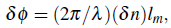where lm is the length over which index change is induced by the applied voltage. The index change δn is proportional to the applied voltage, which is chosen such that δφ = π. Thus, a phase shift of π can be imposed on the optical carrier by applying the required voltage for the duration of each “1” bit.

Semiconductors can also be used to make phase modulators, especially if a multi-quantum-well (MQW) structure is used. The electrorefraction effect originating from the quantum-confinement Stark effect is enhanced for a quantum-well design. Such MQW phase modulators have been developed and are able to operate at a bit rate of up to 40 Gb/s in the wavelength range 1.3-1.6um.

Already in 1992, MQW devices had a modulation bandwidth of 20 GHz and required only 3.85V for introducing a π phase shift when operated near 1.55um. The operating voltage was reduced to 2.8V in a phase modulator based on the electroabsorption effect in a MQW waveguide.

A spot-size converter is sometimes integrated with the phase modulator to reduce coupling losses. The best performance is achieved when a semiconductor phase modulator is monolithically integrated within the transmitter. Such transmitters are quite useful for coherent lightwave systems.

The use of PSK format requires that the phase of the optical carrier remain stable so that phase information can be extracted at the receiver without ambiguity. This requirement puts a stringent condition on the tolerable linewidths of the transmitter laser and the local oscillator.

The linewidth requirement can be somewhat relaxed by using a variant of the PSK format, known as differential phase-shift keying (DPSK). In the case DPSK, information is coded by using the phase difference between two neighboring bits. For instance, if φk represents the phase of the kth bit, the phase difference Δφ = φk – φk-1 is changed by π or 0, depending on whether kth bit is a 1 or 0 bit.

The advantage of DPSK is that the transmittal signal can be demodulated successfully as long as the carrier phase remains relatively stable over a duration of two bits.

#### 3. FSK Format

In the case of FSK modulation, information is coded on the optical carrier by shifting the carrier frequency ω0 itself. For a binary digital signal, ω0 takes two values, ω0 + Δω and ω0 – Δω, depending on whether a 1 or 0 bit is being transmitted.

The shift Δf = Δω/2π is called the frequency deviation. The quantity 2Δf is sometimes called tone spacing, as it represents the frequency spacing between 1 and 0 bits.

The optical field for FSK format can be written as

(Equation 2.3)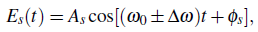where + and – signs correspond to 1 and 0 bits.

By noting that the argument of the cosine can be written as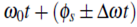, the FSK format can also be viewed as a kind of PSK modulation such that the carrier phase increases or decreases linearly over the bit duration.

The choice of the frequency deviation Δf depends on the available bandwidth. The total bandwidth of a FSK signal is given approximately by 2Δf + 2B, where B is the bit rate.

When Δf >> B, the bandwidth approaches 2Δf and is nearly independent of the bit rate. This case is often referred to as wide-deviation or wideband FSK.

In the opposite case of Δf << B, called narrow-deviation or narrowband FSK, the bandwidth approaches 2B.

The ratio βFM = Δf/B, called the frequency modulation (FM) index, serves to distinguish the two cases, depending on whether βFM >> 1 or βFM << 1.

The implementation of FSK requires modulators capable of shifting the frequency of the incident optical signal. Electro-optic materials such as LiNbO3 normally produce a phase shift proportional to the applied voltage. They can be used for FSK by applying a triangular voltage pulse (sawtooth-like), since a linear phase change corresponds to a frequency shift.

An alternative technique makes use of Bragg scattering from acoustic waves. Such modulators are called acousto-optic modulators. Their use is somewhat cumbersome in the bulk form. However, they can fabricated in compact form using surface acoustic waves on a slab waveguide. The device structure is similar to that of an acousto-optic filter used for wavelength-division multiplexing (WDM) applications. The maximum frequency shift if typically limited to below 1 GHz for such modulators.

The simplest method of producing an FSK signal makes use of the direct-modulation capability of semiconductor lasers. As discussed before, a change in the operating current of a semiconductor laser leads to changes in both the amplitude and frequency of emitted light. In the case of ASK, the frequency shift or chirp of the emitted optical pulse is undesirable. But the same frequency shift can be used to advantage for the purpose of FSK. Typically values of frequency shifts are ~ 1GHz/mA. Therefore, only a small change in the operating current (~1mA) is required for producing the FSK signal. Such current changes are small enough that the amplitude does not change much from bit to bit.

For the purpose of FSK, the FM response of a distributed feedback (DFB) laser should be flat over a bandwidth equal to the bit rate. As seen in figure 3 below, most DFB lasers exhibit a dip in their FM response at a frequency near 1 MHz. The reason is that that two different physical phenomena contribute to the frequency shift when the device current is changed. Changes in the refractive index, responsible for the frequency shift, can occur either because of a temperature shift or because of a change in the carrier density. The thermal effects contribute only up to modulation frequencies of about 1MHz because of their slow response. The FM response decreases in the frequency range 0.1 – 10MHz because of thermal contribution and the carrier-density contribution occur with opposite phases.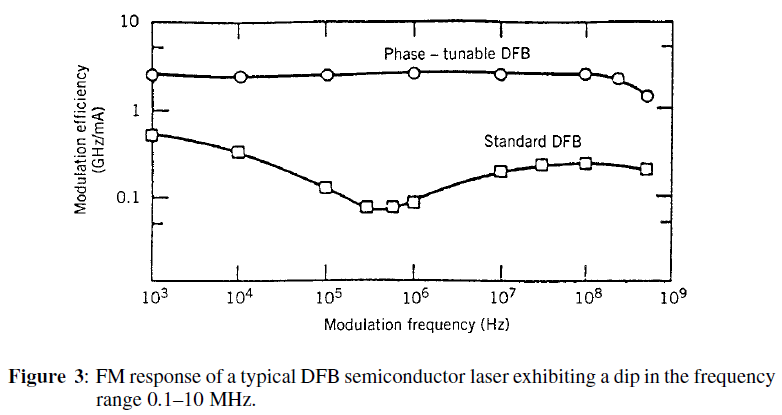Several techniques can be used to make the FM response more uniform. An equalization circuit improves uniformity but also reduces the modulation efficiency. Another technique makes use of transmission codes which reduce the low-frequency components of the data where distortion is highest. Multi-section DFB lasers have been developed to realize a uniform FM response. Figure 3 shows the FM response of a two-section DFB laser. It is not only uniform up to about 1 GHz, but its modulation efficiency is also high. Even better performance is realized by using three-section DBR lasers.

Flat FM response from 100 kHz to 15 GHz was demonstrated in 1990 in such lasers. By 1995, the use of gain-coupled, phase-shifted, DFB lasers extended the range of uniform FM response from 10 kHz to 20 GHz. When FSK is performed through direct modulation, the carrier phase varies continuously from bit to bit. This case is often referred to as continuous-phase FSK (CPFSK). When the tone spacing 2Δf is chosen to be B/2 (βFM = 1/2), CPFSK is also called minimum-shift keying (MSK).

### Demodulation Schemes

As discussed above, either homodyne or heterodyne detection can be used to convert the received optical signal into an electrical form.

In the case of homodyne detection, the optical signal is demodulated directly to the baseband. Although simple in concept, homodyne detection is difficult to implement in practice, as it requires a local oscillator whose frequency matches the carrier frequency exactly and whose phase is locked to the incoming signal. Such a demodulation scheme is called synchronous and is essential for homodyne detection. Although optical phase-locked loops have been developed for this purpose, their use is complicated in practice.

Heterodyne detection simplifies the receiver design, as neither optical phase locking nor frequency matching of the local oscillator is required. However, the electrical signal oscillates rapidly at microwave frequencies and must be demodulated from the IF band to the baseband using techniques similar to those developed for microwave communication systems.

Demodulation can be carried out either synchronously or asynchronously. Asynchronous demodulation is also called incoherent in the radio communication literature. In the optical communication literature, the term coherent detection is used in a wider sense. A lightwave system is called coherent as long as it uses a local oscillator irrespective of the demodulation technique used to convert the IF signal to baseband frequencies.

We will focus on the synchronous and asynchronous demodulation schemes for heterodyne systems.

#### 1. Heterodyne Synchronous Demodulation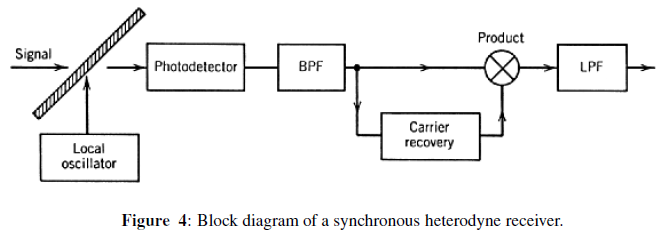Figure 4 shows a synchronous heterodyne receiver schematically. The current generated at the photodiode is passed through a bandpass filter (BPF) centered at the intermediate frequency ωIF. The filtered current in the absence of noise can be written as

(Equation 3.1)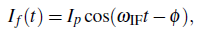where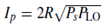and φ = φLO −φs is the phase difference between the local oscillator and the signal. The noise is also filtered by the BPF. Using the in-phase and out-of-phase quadrature components of the filtered Gaussian noise, the receiver noise is included through

(Equation 3.2)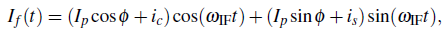where ic and is are Gaussian random variables of zero mean with variance σ2 given by Equation 1.9. For synchronous demodulation, If(t) is multiplied by cos(ωIFt) and filtered by a low-pass filter. The resulting baseband signal is

(Equation 3.3)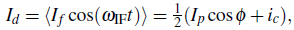where angle brackets denote low-pass filtering used for rejecting the ac components oscillating at 2ωIF. Equation (3.3) shows that only the in-phase noise component affects the performance of synchronous heterodyne receivers.

Synchronous demodulation requires recovery of the microwave carrier at the intermediate frequency ωIF. Several electronic schemes can be used for this purpose, all requiring a kind of electrical phase-locked loop. Two commonly used loops are the squaring loop and the Costas loop. A squaring loop uses a square-law device to obtain a signal of the form cos2IFt) that has a frequency component at 2ωIF. This component can be used to generate a microwave signal at ωIF.

#### 2. Heterodyne Asynchronous Demodulation

Figure 5 below shows an asynchronous heterodyne receiver schematically. It does not require recovery of the microwave carrier at the intermediate frequency, resulting in a much simpler receiver design. The filtered signal If(t) is converted to the baseband by using an envelope detector, followed by a low-pass filter.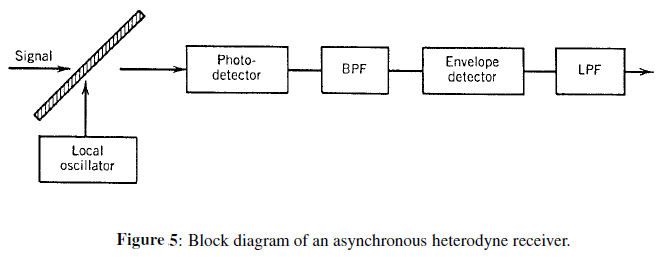The signal received by the decision circuit is just Id = |If |, where If is given by Eq. (3.2). It can be written as

(Equation 3.4)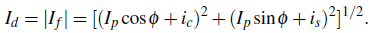The main difference is that both the in-phase and out-of-phase quadrature components of the receiver noise affect the signal. The SNR is thus degraded compared with the case of synchronous demodulation. As discussed, sensitivity degradation resulting from the reduced SNR is quite small (about 0.5 dB). As the phase-stability requirements are quite modest in the case of asynchronous demodulation, this scheme is commonly used for coherent lightwave systems.

The asynchronous heterodyne receiver shown in Fig. 5 requires modifications when the FSK and PSK modulation formats are used.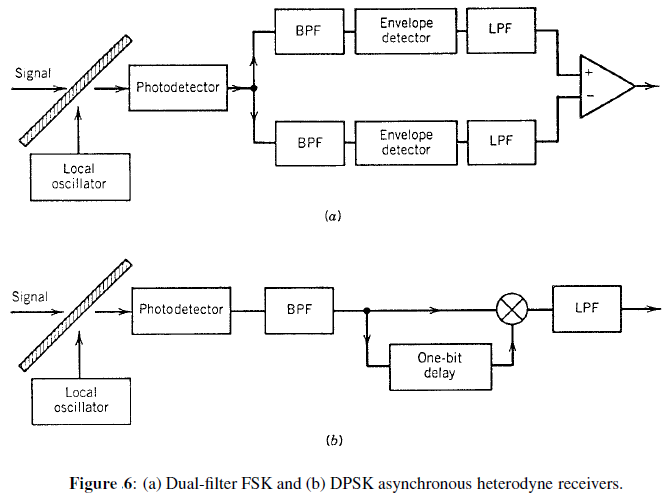Figure 6 shows two demodulation schemes. The FSK dual-filter receiver uses two separate branches to process the 1 and 0 bits whose carrier frequencies, and hence the intermediate frequencies, are different. The scheme can be used whenever the tone spacing is much larger than the bit rates, so that the spectra of 1 and 0 bits have negligible overlap (wide-deviation FSK). The two BPFs have their center frequencies separated exactly by the tone spacing so that each BPF passes either 1 or 0 bits only.

The FSK dual-filter receiver can be thought of as two ASK single-filter receivers in parallel whose outputs are combined before reaching the decision circuit. A single-filter receiver of Fig.  can be used for FSK demodulation if its bandwidth is chosen to be wide enough to pass the entire bit stream. The signal is then processed by a frequency discriminator to identify 1 and 0 bits. This scheme works well only for narrow-deviation FSK, for which tone spacing is less than or comparable to the bit rate (βFM ≤ 1).

Asynchronous demodulation cannot be used in the case the PSK format because the phase of the transmitter laser and the local oscillator are not locked and can drift with time. However, the use of DPSK format permits asynchronous demodulation by using the delay scheme shown in Fig. 6(b).

The idea is to multiply the received bit stream by a replica of it that has been delayed by one bit period. The resulting signal has a component of the form cos(φk − φk−1), where φk is the phase of the kth bit, which can be used to recover the bit pattern since information is encoded in the phase difference φk− φk−1. Such a scheme requires phase stability only over a few bits and can be implemented by using DFB semiconductor lasers. The delay-demodulation scheme can also be used for CPFSK. The amount of delay in that case depends on the tone spacing and is chosen such that the phase is shifted by π for the delayed signal.